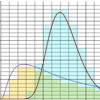#### You may also like### Understanding HypothesesCan you work out the means of these distributions using numerical methods?

# Stats Statements

##### Age 16 to 18Challenge Level
Are the following statistical statements sometimes, always or never true? If they are only sometimes true, can you give examples or conditions under which they are true and under which they are false? Is there some meaningful sense in which they might be 'usually' true or false? Be sure to be clear about your statistical assumptions in each case, especially in those which have a real-life basis.
1. Half of the students taking a test score less than the average mark.

2. Nobody scores higher than the average mark in a test.

3. In a large population of animals, about half of the adult animals are heavier than the average adult weight.

4. Suppose that in a game you can only score an even number of points: 0, 2, 10, 50. So, the average score over a series of games is an even number.

5. A random process is defined by a certain (unknown) probability distribution. The standard deviation of the random process is not larger than the range of the observed data.

6. A random process is defined by a certain probability distribution. The standard deviation of the random process is not larger than half of the maximum theoretical range of the observed data.

7. The chance of observing an outcome more than three standard deviations from the mean is less than 1 in 100.

8. I repeat an experiment with a random numerical outcome many times. Eventually the average of my outcomes will be within 1% of the theoretical average outcome.

9. The chance of observing an outcome more than ten standard deviations from the mean is not more than 1%.

10. If two statistical processes are uncorrelated then they must be independent.

You can view these on cards if you like.

This resource is part of the collection Statistics - Maths of Real Life

NOTES AND BACKGROUND

Statistics is full of many powerful results, but also full of many traps for the unwary. One of the main challenges in becoming a successful statistician is to understand how to perform calculations correctly in situations which seem either obvious or confusing. Knowledge of a statistical technique does not necessarily confer knowledge about when that technique can meaningfully be used! Statisticians are very careful in the language used to set up problems; this requires particular care when assessing whether events are likely or not likely to occur.

In very advanced statistics, mathematicians use the fascinating concept of 'almost surely'. An event will not occur 'almost surely' if the event 'could' occur in principle, but the probability of the event happening is exactly zero. Whilst this might sound impossible, it can occur in a situation in which there is a continuum of possibilities. To visualise this, imagine throwing an infinitely fine dart at a number line. What is the chance of hitting the exact value of $\pi$? Could you hit $\pi$ in principle?